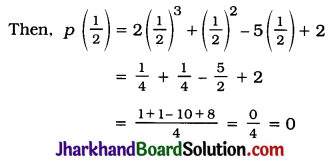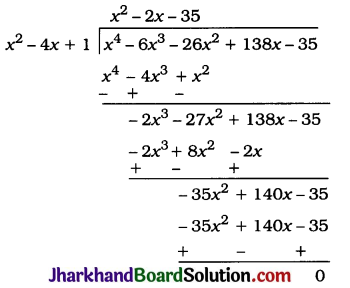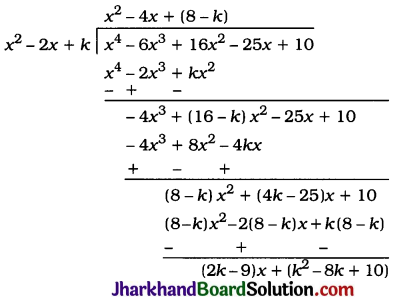# JAC Class 10 Maths Solutions Chapter 2 Polynomials Ex 2.4

Jharkhand Board JAC Class 10 Maths Solutions Chapter 2 Polynomials Ex 2.4 Textbook Exercise Questions and Answers.

## JAC Board Class 10 Maths Solutions Chapter 2 Polynomials Exercise 2.4

Question 1.
Verify that the numbers given alongside of the cubic polynomials below are their zeroes. Also verify the relationship between the zeroes and the coefficients in each case:
1. 2x3 + x2 – 5x + 2; $$\frac{1}{2}$$, 1, -2
2. x3 – 4x2 + 5x – 2; 2, 1, 1
Solution:
1. Let p(x) = 2x3 + x2 – 5x + 2Hence, $$\frac{1}{2}$$ is a zero of
p(x) = 2x3 + x2 – 5x + 2.
Again,
p(1) = 2(1)3 + (1)2 – 5(1) + 2
= 2 + 1 – 5 + 2 = 0
Hence, 1 is a zero of p(x) = 2x3 + x2 – 5x + 2.
Again,
p(-2) = 2(-2)2 + (-2)2 – 5(-2)+2
= -16 + 4 + 10 + 2 = 0
Hence, -2 is a zero of
p(x) = 2x3 + x2 – 5x + 2.
Now, for p(x) = 2x3 + x2 – 5x + 2;
a = 2, b = 1, c = -5 and d = 2.
The zeroes of p (x) are α = $$\frac{1}{2}$$, β = 1 and γ = -2.
Now, α + β + γ = $$\frac{1}{2}$$ + 1 + (-2)
= $$-\frac{1}{2}=\frac{-(1)}{2}=\frac{-b}{a}$$
αβ + βγ + γα = ($$\frac{1}{2}$$) (1) + (1) (-2) + (-2) ($$\frac{1}{2}$$)
= $$\frac{1}{2}$$ – 2 – 1 = $$\frac{-5}{2}=\frac{c}{a}$$ and
αβγ = ($$\frac{1}{2}$$)(1)(-2) = -1 = $$\frac{-(2)}{(2)}=\frac{-d}{a}$$

2. Let p (x) = x3 – 4x2 + 5x – 2.
Then, p (2) = (2)3 – 4(2)2 + 5(2) – 2
= 8 – 16 + 10 – 2 = 0
Hence, 2 is a zero of p (x) = x3 – 4x2 + 5x – 2.
Again,
p(1) = (1)3 – 4(1)2 + 5(1) – 2
= 1 – 4 + 5 – 2 = 0
Hence, 1 is a repeated zero of p(x) = x3 – 4x2 + 5x – 2.
Now, for p(x) = x3 – 4x2 + 5x – 2, a = 1, b = -4, c = 5 and d = -2.
The zeroes of p (x) are α = 2, β = 1 and γ = 1.
Now,
α + β + γ = 2 + 1 + 1 = 4 = $$\frac{-(-4)}{1}=\frac{-b}{a}$$
αβ + βγ + γα = (2) (1) + (1) (1) + (1) (2)
= 2 + 1 + 2 = 5 = $$\frac{5}{1}=\frac{c}{a}$$ and
αβγ = (2) (1) (1) = 2 = $$\frac{-(-2)}{1}=\frac{-d}{a}$$Question 2.
Find a cubic polynomial with the sum of its zeroes, sum of the product of its zeroes taken two at a time, and the product of its zeroes as 2, 7, 14 respectively.
Solution:
Let p (x) = ax3 + bx2 + cx + d, a ≠ 0, be the required cubic polynomial and its zeroes be α, β and γ
Then, as given in the data,
α + β + γ = 2 ∴ $$\frac{-b}{a}$$ = 2
αβ + βγ + γα = -7 ∴ $$\frac{c}{a}$$ = -7
αβγ = $$\frac{-d}{a}$$ = -14
So, if a = 1, then b = -2, c = -7 and d = 14.
Hence, the required polynomial is p(x) = x3 – 2x2 – 7x + 14.

Question 3.
If the zeroes of the polynomial x3 – 3x2 + x + 1 are a – b, a, a + b, find a and b.
Solution:
For the given polynomial x3 – 3x2 + x + 1.
A = 1, B = -3, C = 1 and D = 1.
Its zeroes are given to be a – b, a and a + b.
Now, sum of zeroes = (a – b) + a + (a + b) = 3a
From the polynomial,
Sum of zeroes = $$\frac{-B}{A}=\frac{-(-3)}{1}=3$$
Hence, 3a = 3 ∴ a = 1
Product of zeroes = (a – b) × a × (a + b)
= a(a2 – b2)
From the polynomial.
Product of zeroes = $$\frac{-\mathrm{D}}{\mathrm{A}}=\frac{-1}{1}=-1$$
Hence, a (a2 – b2) = -1
1(12 – b2) = -1 ∴ 1 – b2 = -1
∴ 1 + 1 = b2 ∴ b2 = 2
∴ b = ±$$\sqrt{2}$$
Thus, a = 1 and b = ±$$\sqrt{2}$$.

Question 4.
It two zeroes of the polynomial x4 – 6x3 – 26x2 + 138x – 35 are 2±$$\sqrt{3}$$, find other zeroes.
Solution:
Let p (x) = x4 – 6x3 – 26x2 + 138x – 35
2 + $$\sqrt{3}$$ and 2 – $$\sqrt{3}$$ are zeroes of p (x).
∴ (x – 2 – $$\sqrt{3}$$)(x – 2 + $$\sqrt{3}$$) = (x – 2)2 – ($$\sqrt{3}$$)2
= x2 – 4x + 4 – 3
= x2 – 4x + 1
is a factor of p(x).Then, Quotient = x2 – 2x – 35
= x2 – 7x + 5x – 35
= x(x – 7) + 5(x – 7)
=(x – 7)(x + 5)
x – 7 = 0 gives x = 7 and x + 5 = 0 gives x = -5.
Hence, the other zeroes of the given polynomial are 7 and -5.Question 5.
If the polynomial x4 – 6x3 + 16x2 – 25x + 10 is divided by another polynomial x2 – 2x + k, the remainder comes out to be x + a, find k and a.
Solution:But, the remainder is given to be x + a.
Hence, comparing the coefficients of x and the constant term, we get
2k – 9 = 1 and k2 – 8k + 10 = a
Now, 2k – 9 = 1
∴ 2k = 10
∴ k = 5
and a = k2 – 8k + 10
∴ a = (5)2 – 8(5) + 10
∴ a = 25 – 40 + 10
∴ a = -5
Thus, k = 5 and a = -5.